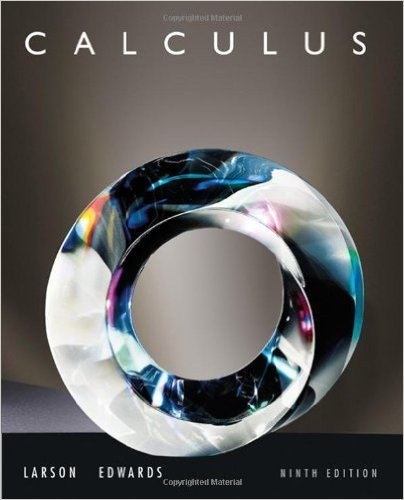×
×

# Solutions for Chapter P.S.: Integration## Full solutions for Calculus | 9th Edition

ISBN: 9780547167022Solutions for Chapter P.S.: Integration

Solutions for Chapter P.S.
4 5 0 385 Reviews
13
5
##### ISBN: 9780547167022

This expansive textbook survival guide covers the following chapters and their solutions. Chapter P.S.: Integration includes 21 full step-by-step solutions. This textbook survival guide was created for the textbook: Calculus , edition: 9. Since 21 problems in chapter P.S.: Integration have been answered, more than 67681 students have viewed full step-by-step solutions from this chapter. Calculus was written by and is associated to the ISBN: 9780547167022.

Key Calculus Terms and definitions covered in this textbook
• Amplitude

See Sinusoid.

• Arccosecant function

See Inverse cosecant function.

• Composition of functions

(f ? g) (x) = f (g(x))

• Deductive reasoning

The process of utilizing general information to prove a specific hypothesis

• Dihedral angle

An angle formed by two intersecting planes,

• Equally likely outcomes

Outcomes of an experiment that have the same probability of occurring.

• Expanded form of a series

A series written explicitly as a sum of terms (not in summation notation).

• Extracting square roots

A method for solving equations in the form x 2 = k.

• Geometric series

A series whose terms form a geometric sequence.

• Horizontal asymptote

The line is a horizontal asymptote of the graph of a function ƒ if lim x:- q ƒ(x) = or lim x: q ƒ(x) = b

• Implicitly defined function

A function that is a subset of a relation defined by an equation in x and y.

• Left-hand limit of f at x a

The limit of ƒ as x approaches a from the left.

• Length of an arrow

See Magnitude of an arrow.

• Magnitude of an arrow

The magnitude of PQ is the distance between P and Q

• Negative angle

Angle generated by clockwise rotation.

• Parallel lines

Two lines that are both vertical or have equal slopes.

• Pseudo-random numbers

Computer-generated numbers that can be used to approximate true randomness in scientific studies. Since they depend on iterative computer algorithms, they are not truly random

• Right triangle

A triangle with a 90° angle.

• Ymax

The y-value of the top of the viewing window.

• Zero of a function

A value in the domain of a function that makes the function value zero.

×